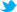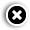# Microsoft introduces Math Solver app, uses AI to solve problems

## Microsoft Math App: The Redmond-based tech company has introduced an AI-based Math Solver Application. Here's all about the appNidhi Singal | January 17, 2020 | Updated 15:23 ISTThis app is available both on Android and iOS.

Microsoft is making solving mathematic problems a little easier. The Redmond-headquartered technology giant has introduced an AI-based Math Solver application that can be used to solve math problems on a smartphone or a tablet. Microsoft says that the app can be used to help with a wide range of mathematical concepts-from elementary arithmetic and quadratic equations to calculus and statistics.

Available for iOS and Android platform, the user can use the smartphone camera to capture an image of the mathematical problem or can even scribble the math problem on the smartphone or the tablet's display.

How does it work?

According to Microsoft, the Math Solver uses Artificial Intelligence (AI) to instantly recognise the problem and deliver an accurate solution. The app can also be used as a mathematical learning aid for students as it will also provide a step-by-step explanation with additional learning materials such as worksheets and video tutorials. It also provides interactive graphs to help learners understand the correlation. On the app, users can scan and plot X-Y data tables for linear or non-linear functions. With interactive definitions and explanations, students can easily grasp and retain concepts better.

Supported problems on the Math Solver app include elementary (arithmetic, real, complex numbers, LCM, GCD, factors, roman numerals), pre-algebra (radicals and exponents, fractions, matrices, determinants), algebra (quadratic equations, system of equations, inequalities, rational expressions, linear, quadratic and exponential graphs), basic calculus (summations, limits, derivatives, integrals), statistics (mean, median, mode, standard deviation, permutations, combinations) and word problems on math concepts, number theory, probability, volume, surface area.

How to use?

Interestingly, the Math Solver application supports a wide range of inputs - draw, scan or type. A user can simply draw on the device's display with a finger or use a stylus. As the application is AI-powered, it will recognise the handwriting and show the problem instantly in text form. The second method is to capture the photo of the equation and upload the image in the application. Alternatively, there is a provision to type the problem statement, using the scientific calculator in the app.

The app has a neat user interface with the scan, draw and type option on the centre top. On the top left is the notes icon that shows the list of problems that a user can try. This includes problems across fractions, rational equation, radical equation, logarithmic equation, arithmetic, trigonometry, differentiation and more.

Which languages does it support?

Microsoft's Math Solver application supports a wide range of languages. Of the 22 languages support, it includes 12 Indian languages namely Assamese, Bengali, Gujarati, Hindi, Kannada, Konkani, Marathi, Malayalam, Oriya, Punjabi, Tamil, and Telugu. Some of the international languages supported include German, Spanish, Simplified Chinese, and Russian.

• Print
• COMMENT
##### Xiaomi to launch Mi Mix Alpha with wrap-around display in India soon